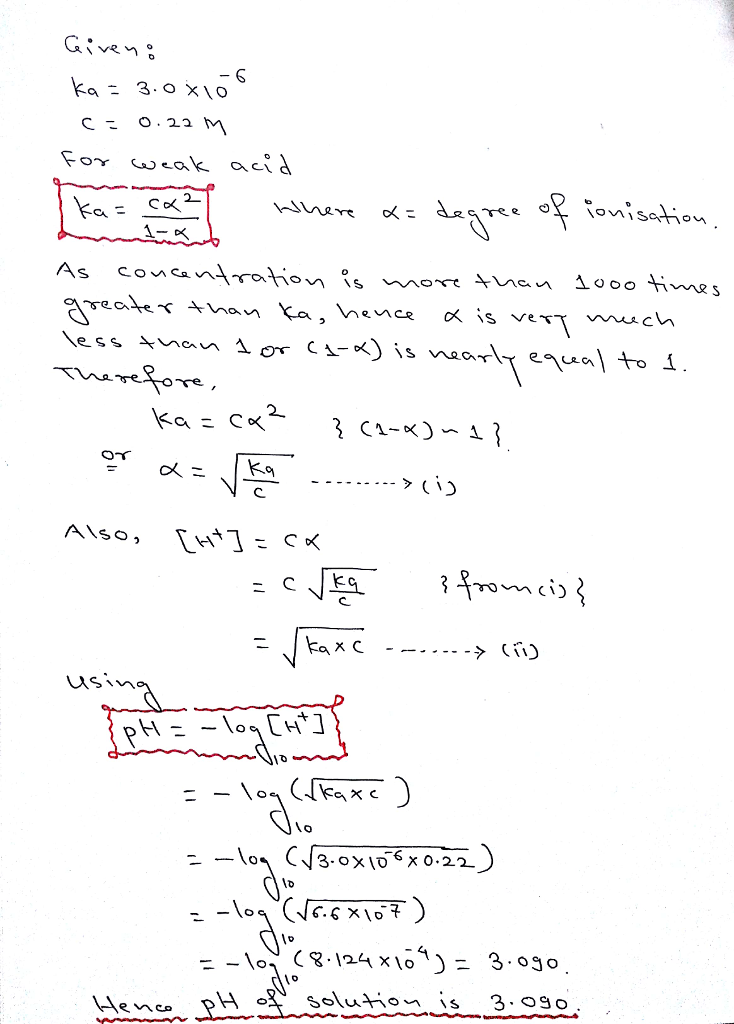# Question & Answer: The K_a of a monoprotic weak acid is 3.0 times 10^-6, what is the pH of a 0.22 M solution of…..

The K_a of a monoprotic weak acid is 3.0 times 10^-6, what is the pH of a 0.22 M solution of this acid? pH = If the concentration is more than 1000 times greater than the K_a, then you can make an assumption that simplifies the algebra needed to solve the problem. Otherwise, you need to solve a quadratic equation.

Answer